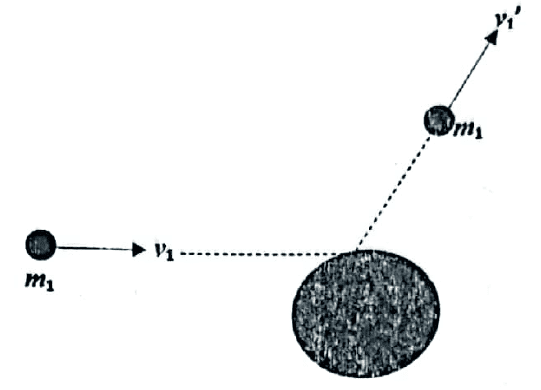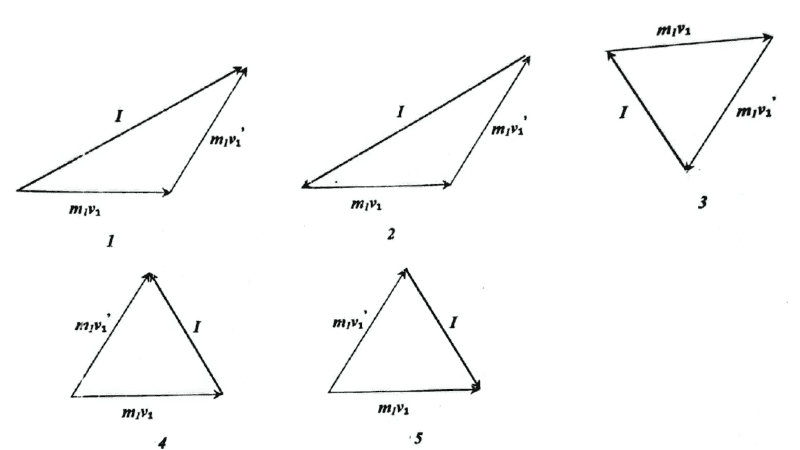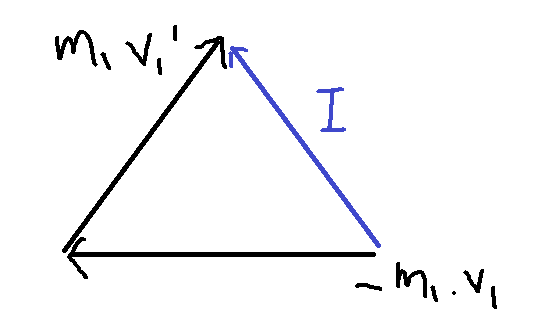# Vector Diagram of Impulse

• songoku

#### songoku

Homework Statement
Object ##m_1## moves with velocity ##v_1## collides with object ##m_2## and has final velocity of ##v_{1}^{'}##. Which vector diagram is correct?
Relevant Equations
I = ΔpI think all the options are wrong. Since I = Δp = m1v1' - m1v1, I draw it like this:Is my drawing wrong?

Thanks

Your diagram is not different from one of the 5 choices (which one?) in the same manner that ##A - B## is not different from ##A + (-B).##

•songoku
At times, I think it is better to think of ##\Delta \vec p=\vec p_f - \vec p_i##
implicitly but "physically"
as what has to be added to ##\vec p_i## to get ##\vec p_f##: $$\vec p_i + \Delta \vec p= \vec p_f$$

•songoku and Orodruin
Thank you very much kuruman and robphy

From conservation of momentum, I would be looking for the diagram in which the vector representing ##m_1v_1## is the vector sum of the other two.

Homework Statement:: Object ##m_1## moves with velocity ##v_1## collides with object ##m_2## and has final velocity of ##v_{1}^{'}##. Which vector diagram is correct?
Relevant Equations:: I = Δp
The question here is which ##\vec{\Delta p}## ? ##\vec{\Delta p}## experienced by ##m_2## or ##\vec{\Delta p}## experienced by ##m_1## ? Because they are equal and opposite.

Edited to show ##\vec{\Delta p}## as a vector.

Last edited:
•songoku
The question here is which ##\Delta p## ? ##\Delta p## experienced by ##m_2## or ##\Delta p## experienced by ##m_1## ? Because they are equal and opposite.
I too considered this ambiguity. I decided that it is the Δp of the object whose initial and final momenta are shown because the initial and final momenta of the target mass could be anything. I classified this as a vector subtraction problem.

•songoku and neilparker62
Then the correct option is the one showing ##\vec{p_1} + \vec{\Delta p} = \vec{p_1}'## with ##\vec{\Delta p}## being the collision impulse experienced by mass ##m_1##. In which case I stand corrected in respect of post #5 in which I assumed ##\vec{\Delta p}## is the collision impulse experienced by (also) assumed stationary object ##m_2##. Tricky!

•songoku